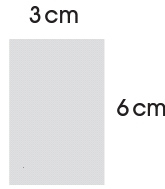# Third Term Examination Mathematics Primary 3 (Basic 3) – Exam Questions

MATHEMATICS

THIRD TERM EXAMINATION

PRIMARY 3 (BASIC 3)

SECTION A

Attempt all the questions.

Choose the correct answer from the options.

1. The area of a rectangle is given by the formula __________.

[a] gram x width

[c] 1/2 x base x height

2. Calculate the perimeter of a square of side 9m.

[a] 36 m

[b] 18 m

[c] 81 m

3. Which of these is not a unit of measurement?

[a] Centimetres

[b] Grams

[c] Kilograms

4. A rectangle measures 7cm by 4cm, what is the perimeter?

[a] 22 cm

[b] 28 cm

[c] 11 cm

5. How many grams are there in 1 kilogram?

[a] 10

[b] 1000

[c] 100

6. Subtract 500 ml from 250 ml.

[a] 280 ml

[b] 250 ml

[c] 240 ml

7. Which of these objects is the heaviest?

[a]

[b]

[c]

8. __________ gives more accurate measures.

[a] Non standard

[b] Standard measures

[c] None of the above

9. Capacity is measured in __________.

[a] kilogram

[b] gram

[c] litre

10. A line of symmetry is any line that __________.

[a] is curvy

[b] divides a shape into two equal part

[c] mark out a shape

11. Find the mode: 36, 44, 32, 48, 46, 32, 45, 33

[a] 48

[b] 36

[c] 32

12. Akin ran from 6 o’ clock until quarter past 6. How many minutes did he run?

[a] 30 minutes

[b] 15 minutes

[c] 10 minutes

13. Which of these is equal to 36?

[a] 3 x 5 x 7

[b] 2 x 2 x 3 x 3

[c] 2 x 2 x 2

14. Chinedu’s shop sells 35 kg of sugar per month. How much sugar will he sell in 7 month?

[a] 100 kg

[b] 200 kg

[c] 245 kg

15. What says the time?

[a] quarter past 9

[b] half past 9

[c] 9 o’ clock

Get more Mathematics Exam Questions – Third Term Examination Mathematics Link

SECTION B

Attempt all questions in this section.

QUESTION 1

Work out the area of each shape:

I.II.B. Find the perimeter of the following shapes.

I.II.QUESTION 2

A. Grandma is 79 years old. Grandpa is 10 years older than grandma. How old is grandpa?

B. Mom uses 15 litres of kerosene every week when she prepares food. How much kerosene does she use in 5 weeks?

QUESTION 3

Draw clock faces and fill in these times.

A. Quarter past 11

B. 6 o’ clock

QUESTION 4

A. Draw these shapes:

I. Cone

II. Cube

B. Draw a line of symmetry.QUESTION 5

Number of pupils in a class each day.

Monday   Tuesday   Wednesday   Thursday   Friday

30              27                33                  26          20

Draw a pictogram to represent the data.

1.2.3.4.5.